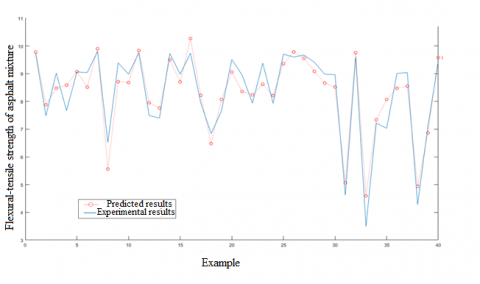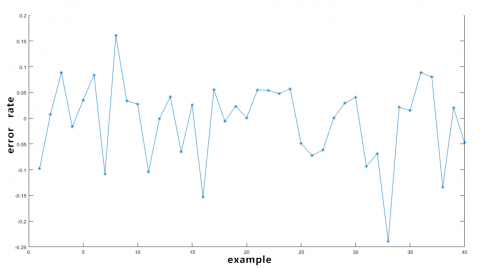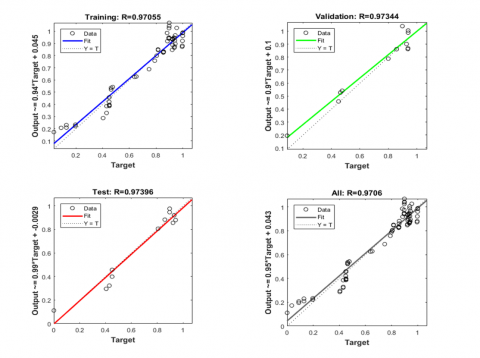# A Backpropagation Neural Network-based Flexural-tensile Strength Prediction Model for Asphalt Mixture in Cold Regions under Cyclic Thermal Stress

A Backpropagation Neural Network-based Flexural-tensile Strength Prediction Model for Asphalt Mixture in Cold Regions under Cyclic Thermal Stress
College of Transportation, Jilin University, Changchun 130021, China

College of Construction Engineering, Jilin University, Changchun 130026, China

Corresponding Author Email:
tanyp17@mails.jlu.edu.cn
Page:
433-436
|
DOI:
https://doi.org/10.18280/mmep.060315
18 May 2019
|
Accepted:
20 July 2019
|
Published:
15 September 2019
| Citation

OPEN ACCESS

Abstract:

This paper attempts to disclose how the varying cold season temperature affects the performance of asphalt mixture i=n cold regions, and create a model to predict the flexural-tensile strength under cyclic thermal stress. For this purpose, the author investigated the influencing factors of asphalt mixture performance in cold regions, such as temperature level and variation in temperature difference, and employed the backpropagation neural network (BPNN) to learn, train and verify 120 samples of SBS AC-13 database. On this basis, a BPNN-based prediction model was established for the flexural-tensile strength of asphalt mixture under cyclic thermal stress. Next, the predicted results were compared with the actual results through regression analysis. The comparison shows that our model output a correlation coefficient (R) of 0.9706, an evidence for good prediction accuracy. This means our model can effectively predict the flexural-tensile strengths of asphalt mixture under cyclic thermal stress in cold regions. The research findings provide a good reference for similar studies on asphalt mixture.

Keywords:

cold regions, asphalt mixture, flexural-tensile strength, backpropagation neural network (BPNN), regression analysis

1. Introduction

In the cold regions of China, the annual average temperature is as low as -2 °C, and the highest temperature is not more than 7 °C. The low temperature has brought a 7~8 month-long freezing period each year. In winter, the outdoor temperature often changes rapidly, exerting a great impact on asphalt concrete pavement. The impact is particularly prominent in northern China, where there is a big temperature difference between winter and summer. Under the rapid variation in temperature, the thin layers of the asphalt pavement will face a high thermal stress, and suffer from fatigue cracking induced by temperature. To evaluate the flexural-tensile performance of asphalt mixture in China’s cold regions, it is necessary to build a flexural-tensile strength prediction model for asphalt in such regions under cyclic thermal stress [1-3].

The neural network (NN) has been widely applied to analyze the performance of asphalt mixture. For example, Cui Pingde, Yang Fu et al. adopted the NN to forecast and discuss the shear strength of asphalt mixture. Liu Xiaoming et al. established an NN model to predict the elastic modulus of emulsified asphalt mixture. However, there is no report on the flexural-tensile strength changes of asphalt mixture in cold regions through the cold season .

This paper carries out in-depth learning and training of the existing experimental data with backpropagation neural network (BPNN), establishes a flexural-tensile strength prediction model under cyclic thermal stress, and measures the actual error between the predicted and actual results. Next, the flexural-tensile strength of asphalt mixture under cyclic thermal stress was subjected to multivariate regression analysis, and the error between the training sample and the training output was identified. The results show that the nonlinear mapping relationship of the established BPNN-based prediction model can accurately simulate how the flexural-tensile strength of asphalt mixture varies with the multiple variables and forecast its future trend.

2. Introduction to the BPNN

The BPNN is a multi-layer feedforward network trained by the error backpropagation algorithm. It was proposed in 1986 as an advanced NN. Since then, the BPNN has become the most popular NN, thanks to its excellence in solving problems like regression prediction and pattern recognition .

The BPNN is made up of multiple adaptive simple neurons in parallel connection. During network training, the connection weights and biases are updated in real time according to a fixed number of relatively stable training samples, such that the error of the output continuously declines and converges to the desired output. The trained network will be able to predict the actual output of the test samples .

In general, a BPNN consists of an input layer, a hidden layer and an output layer. Each layer contains one or more nodes. For each node, the relationship between its input and output depends on connection weights and input/output threshold .

Suppose there are m input layer nodes (inputs) and k hidden layer nodes (outputs). Then, the input vectors and output vectors can-be-described-as $X=\left(x_{1}, x_{2}, \ldots, x_{j}, \ldots, x_{m}\right)^{T}$ -and $T=\left(y_{1}, y_{2}, \ldots, y_{j}, \ldots, y_{k}\right)^{T},$ 'respectively. Unlike the first two-layers, the output-layer has only one-node. The -computing results of this -layer can be expressed-as $O=\left(o_{1}, 0_{2}, \ldots, 0_{j}, \ldots, 0_{n}\right)^{T},$ while- the desired results can-be depicted as $D=\left(d_{1}, d_{2}, \ldots, d_{j}, \ldots, d_{n}\right)^{T}$ (n is the number of samples being trained). The weight of a connection between the input and hidden layers can be formulated as $V=\left(v_{i j}\right)_{m \times k}$ [7-9], where vij is a matrix element reflecting the weight of the connection between input layer node i and hidden layer node j.

The internodal correspondence effectively characterizes the nonlinear mapping property of the BPNN, enabling the network to disclose the mechanism and principle of complex nonlinear problems .

3. Model Construction and Application

3.1 Sample sets

To prevent the PNN from over-fitting, this paper attempts to control the number of sample trainings. Firstly, several samples were divided into a training set and a validation set. The former was used to correct the connection weights, adjust the input/output threshold and determine the suitable gradient, while the latter is responsible for regulating and training the error in real time . Then, a program was designed to split the samples randomly into three parts, so that each part contains a reasonable number of samples. Among the three parts, the training set has 80 samples and the testing set has 40.

3.2 Input and output indices

The low-temperature ultimate flexural-tensile strain of small beams is affected by many complex factors. Among them, the asphalt property and mineral gradation are two key determinants of the magnitude of the strain . Hence, these two factors were selected as the main research targets to ensure the computing accuracy and efficiency of the BPNN-based prediction model. In addition, the low-temperature ultimate flexural-tensile strain of small beams was defined as the output of the model.

3.3 Network structure

Being a type of topology model, a three-layer BPNN was selected for our research. In this network, the hidden layer nodes transmit the weights via hyperbolic tangent sigmoid function . This function maps the range of each input layer node (-∞, +∞) to that of each hidden layer node (-1, +1). As a differentiable function, it is suitable for training the samples at the node . The output layer uses the linear transfer function purelin to excite and transfer the m input vectors, yielding a prediction matrix. In addition, the gradient descent algorithm was selected to control the error rate to the required level. This algorithm has been proved through experiment to have better convergence and stability than the other algorithms.

Table 1 lists the number of nodes in each layer. To control the input/output threshold within the interval of [0, 1], the tangent sigmoid function (tansig) was selected as the activation and transfer function between the input and hidden layers, and the linear function (logsig) as the activation of transfer function for output layer nodes . Furthermore, the traingd function was employed to train our NN. This function is often used in negative gradient descent training, which is an accurate and rational way to train the NN. The training was terminated when the number of error cycles of the testing set reached 1,000. The number of input and hidden layer nodes were determined by trial and error .

Table 1. Functions of the BPNN-based prediction model

 Number of layer Number of input layer nodes Number of hidden layer nodes Number of output layer node Training step length Desired error Transfer function of the input layer Transfer function of the output layer Training function 3 2 5 1 1,000 0.0003 tansig purelin traingd
4. Model Verification and Results Analysis

The BPNN learns and analyzes specific problems through training, and adjusts adaptively to formulate reasonable mapping rules between the input and output of each problem. During this process, the weights of the network serve as the carriers of memory and storage. The BPNN can also express the new knowledge acquired through autonomous learning. In this paper, 40 training samples are selected randomly and used to train the NN. The prediction results thus obtained were compared with the actual experimental data. The fitness and error curves are shown in Figure 1 below.

As shown in Figure 1, the predicted results on the 40 samples differed slightly from the experimental results, revealing a high fitness and accuracy of our model in the prediction of flexural-tensile strength of asphalt mixture.

To quantify the error between the predicted and experimental results, the error rate curve of our model was plotted (Figure 2), which shows that the error rate was rather small (20 %) for the testing samples. This means the BPNN-based prediction model has a good fault tolerance, that is, the model can work normally despite local damage or injury of the system.

If the input and output results fall in the interval [0, +1], then the BPNN must have reached the optimal performance. To obtain scientific and accurate simulation results, the sample data were normalized before training and the trained outputs were denormalized by the logarithmic algorithm with preset maximum and minimum. Taking the network output and the desired output as a pair of coordinates (x, y), the prediction effect is better if the value of x approaches that of y. The regression results are not significant unless the (x, y) pairs obtained by nonlinear mapping of the BPNN are distributed across the y=x line. Figure 3 presents the linear regression results of multiple (x, y) pairs in the training phase, the testing phase and the entire prediction period.

It can be seen from Figure 3 that the regression results were significant in both the training phase and the testing phase. The correlation coefficient R of our model to training samples, testing samples and all samples was 97.05 %, 97.39 % and 97.06 %, respectively. All of them were above the required level of 80 %.

To sum up, our BPNN-based prediction model was proved, both quantitatively and qualitatively, as capable of predict the exact flexural-tensile strength of asphalt mixture under cyclic thermal stress.

## f1-15.pngFigure 1. The fitness and error between predicted and experimental results

## f2-15.pngFigure 2. The error rate of our model

## f3-15.pngFigure 3. The regression results of the prediction effect

5. Conclusions

This paper probes deep into the influencing factors of flexural-tensile strength of asphalt mixture under cyclic thermal stress in cold regions. Considering the two most significant influencing factors, the author established a BPNN-based model to predict the flexural-tensile strength of asphalt mixture under cyclic thermal stress. The model enjoys a high fitness thanks to the training by a three-layer BPNN. The experimental results show that our model can preliminarily predict the flexural-tensile strength of asphalt mixture under cyclic thermal stress in the cold season, based on the huge amount of existing experimental data on asphalt mixture. The research findings effectively improve the working efficiency of personnel, the utilization rate of materials and the rationality of budget for asphalt concrete pavements.

Acknowledgement

This paper is supported by National Natural Science Foundation of China (Grant No.: 51508223) and Natural Science Foundation of Jilin Province (Grant No.: 20160101267JC).

References

 Elwardany, M.D., Rad, F.Y., Castorena, C. (2016). Evaluation of asphalt mixture laboratory long-term ageing methods for performance testing and prediction. Road Materials & Pavement Design, 18(sup1): 28-61. https://doi.org/10.1080/14680629.2016.1266740

 Achour, T., El Euch Khay, S., Jarraya, E., Neji, J. (2017). Combined contribution of experiments and modeling to better understand mechanical properties of concrete. Revue des Composites et des Matériaux Avancés, 27(1-2): 123-136. https://doi.org/10.3166/rcma.2017.00008

 Nega, A., Nikraz, H., Leek, C. (2013). Evaluation and validation of characterization methods for fatigue performance of asphalt mixes for Western Australia. Advanced Materials Research, 723: 75-85. https://doi.org/10.4028/www.scientific.net/AMR.723.75

 Ziaei-Rad, V., Nouri, N., Ziaei-Rad, S. (2012). A numerical study on mechanical performance of asphalt mixture using a meso-scale finite element model. Finite Elements in Analysis & Design, 57(57): 81-91. https://doi.org/10.1016/j.finel.2012.03.004

 Kim, Y.R., Hong, M., Allen, D.H. (2013). Statistical and dimensional analysis of hot-mix asphalt mixture characteristics on asphalt pavement analyser rutting behaviour. International Journal of Pavement Engineering, 14(2): 103-115. https://doi.org/10.1080/10298436.2011.633706

 Amini, A., Goli, A., Ziari, H. (2017). The influence of nanoclay on the performance properties and moisture susceptibility of rubberized asphalt mixture. Liquid Fuels Technology, 35(2): 8. https://doi.org/10.1080/10916466.2016.1248775

 Soltani, M., Moghaddam, T.B., Karim, M.R. (2015). Stiffness performance of polyethylene terephthalate modified asphalt mixtures estimation using support vector machine-firefly algorithm. Measurement, 63: 232-239. https://doi.org/10.1016/j.measurement.2014.11.022

 Jeffry, S.N.A., Jaya, R.P., Hassan, N.A. (2018). Mechanical performance of asphalt mixture containing nano-charcoal coconut shell ash. Construction & Building Materials, 173: 40-48. https://doi.org/10.1016/j.conbuildmat.2018.04.024

 Pasetto, M., Baldo, N. (2011). Mix design and performance analysis of asphalt concretes with electric arc furnace slag. Construction & Building Materials, 25(8): 3458-3468. https://doi.org/10.1016/j.conbuildmat.2011.03.037

 Mahmoud, E., Masad, E., Nazarian, S. (2015). Discrete element analysis of the influences of aggregate properties and internal structure on fracture in asphalt mixtures. Journal of Materials in Civil Engineering, 22(1): 10-20. https://doi.org/10.1061/(ASCE)MT.1943-5533.0000005

 Ozgan, E. (2011). Artificial neural network based modelling of the Marshall Stability of asphalt concrete. Expert Systems with Applications, 38(5): 6025-6030. https://doi.org/10.1016/j.eswa.2010.11.018

 Ozturk, H.I., Kutay, M.E. (2014). An artificial neural network model for virtual Superpave asphalt mixture design. International Journal of Pavement Engineering, 15(2): 151-162. https://doi.org/10.1016/j.eswa.2010.11.018

 Wang, Z., Cai, L., Wang, X. (2018). Fatigue performance of different thickness structure combinations of hot mix asphalt and cement emulsified asphalt mixtures. Materials, 11(7): 1145. https://doi.org/10.3390/ma11071145

 Du, S. (2018). Effect of curing conditions on properties of cement asphalt emulsion mixture. Construction & Building Materials, 164: 84-93. https://doi.org/10.1016/j.conbuildmat.2017.12.179

 Zavrtanik, N, Prosen, J, Tušar, M. (2016). The use of artificial neural networks for modeling air void content in aggregate mixture. Automation in Construction, 63: 155-161. https://doi.org/10.1016/j.autcon.2015.12.009